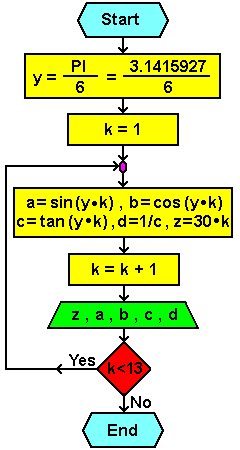# 6.1.4. Flow of trigonometric functions1. phase
Calculation of trigonometric functions.

2. phase
The calculated value of the angle X in the range of 30-360° with a jump of 30°.
For calculated values of the angle calculate the amount of of functions:
sinX, cosX, tgX i ctgX.

3. phase
Mathematical expressions needed in this task are:

PI = 3.1415927
-------------------------------------------------
X = (PI / 6)•k
za k = 0 do 12

a = sinX , b = cosX , c = tgX , d = 1/tgX

4. phase
Flowchart:Citing of this page: Radic, Drago. " IT - Informatics Alphabet " Split-Croatia. {Date of access}. . Copyright © by Drago Radic. All rights reserved. | Disclaimer